Home > GHCN, trb > trb-0.04: Proportional Latitudinal Zoning

## trb-0.04: Proportional Latitudinal Zoning

2010 July 13

Introduction

“A new version, conceived in liberty, and dedicated to the proposition that all latitudinal zones are created equal”

Equal Area Latitudinal Zones

This is the change discussed yesterday which makes each latitudinal zone an equal area. There is a counter-argument for using ‘fixed step’ zones. The temp changes in the high latitudes are expected to occur faster than the low latitudes, so it might make sense to display them in finer resolution (ie … smaller area per zone). And I’m pretty sure that CRU uses a fixed lat zone step for its gridding. Nevertheless, while we know how to do fixed step zones (see trb-0.03), for now, trb will use equal area latitude zones.

Code

```###############################################################################
# trb 0.04 : 2010 07 13 : Proportional Latitudinal Bands
# trb 0.03 : 2010 07 12 : Weighted Latitudinal Bands
# trb 0.02 : 2010 07 11 : Simple Latitudinal Bands
# trb 0.01 : 2010 07 10 : GHCN Simple Mean Average
# https://rhinohide.wordpress.com
###############################################################################

###############################################################################
###############################################################################
# ftp://ftp.ncdc.noaa.gov/pub/data/ghcn/v2/v2.mean
# ftp://ftp.ncdc.noaa.gov/pub/data/ghcn/v2/v2.temperature.inv
###############################################################################

#------------------------------------------------------------------------------
# Temperature File (GHCN formatted monthly means)
#------------------------------------------------------------------------------

# mm for 'monthly means'
mmwids=c(11,1,4,5,5,5,5,5,5,5,5,5,5,5,5)
mmcols=c("id","dupe","year","jan","feb","mar","apr","may","jun",
"jul","aug","sep","oct","nov","dec")

#------------------------------------------------------------------------------
# Inventory File
#------------------------------------------------------------------------------

# there are five occurrances of the pound hash in v2.temperature.inv
# R scripts chokes on reading those, remove them first

invcols <- c("id","name","lat","lon","alt","elev","ru","pop","terr","arid",
invwids <- c(11,32,6,8,5,5,1,5,2,2,2,2,1,2,16,1,5)

###############################################################################
# Set up arrays for latitudinal banding
###############################################################################

hindex=new.env(hash=TRUE)
hlat=new.env(hash=TRUE)
hlon=new.env(hash=TRUE)
for (i in 1:nrow(inv)) {
hindex[[as.character(inv[i,1])]] <- i
hlat[[as.character(inv[i,1])]] <- inv[i,3]
hlon[[as.character(inv[i,1])]] <- inv[i,4]
}

mmlat <- rep(NA,nrow(mm))
mmlon <- rep(NA,nrow(mm))

for (i in 1:nrow(mm)) {
mmlat[i] <- hlat[[as.character(mm[i,1])]]
mmlon[i] <- hlon[[as.character(mm[i,1])]]
}

# one array to rule them all and ...
mm1 <- cbind(mm,mmlat,mmlon, rowMeans(mm[,4-15],na.rm=T))

# for everything there is a season
mm <- NULL
inv <- NULL
mmlat <- NULL
mmlon <- NULL
hlat <- NULL
hlon <- NULL

# general formula for a set number of latitude bands of equal area
nbands <- 8
bands <- rep(NA,nbands)
bands=b
for (i in 1:nbands) {
a = asin(sin(b*pi/180)-2/nbands)
b = a * 180 / pi
bands[i+1] = b
}
bands

###############################################################################
# Calculate Annual Mean Average
###############################################################################

#------------------------------------------------------------------------------
# set up the range of years to calculate
#------------------------------------------------------------------------------
#year0 <- min(mm1[,3])
year0 <- 1880              # 1880 is an arbitrary start date
year1 <- max(mm1[,3])
range <- year1 - year0 + 1

#------------------------------------------------------------------------------
# initialize some vectors to hold annual results
#------------------------------------------------------------------------------
lyear <- rep(NA, range)
lmean <- matrix(NA,range,nbands)
lcount <- matrix(NA,range,nbands)

#------------------------------------------------------------------------------
# loop through the range of years
#------------------------------------------------------------------------------
for (i in 1:range) {

year <- i + year0 -1

#--------------------------------------------------------------------------
# loop through the latitude bands
#--------------------------------------------------------------------------

for (j in 1:nbands) {

# select a latitude band for this year
idx=(mm1[,16]<=bands[j] & mm1[,16]>=bands[j+1] & mm1[,3]==year)
mmlt=mm1[idx,]

# calculate the annual means
# divide by 10 since means are stored as 10ths of T

# fractional area for this latitudinal band
# fa <- abs((sin(bands[j]*pi/180) - sin(bands[j+1]*pi/180)))/2

# don't need fractional area when every band has equal area
fa <- 1

# only use years with data for every month
lmean[i,j]= (mean(rowMeans(mmlt[,4:15]),na.rm=T)/10) * fa

# use every year, even those with partial data
#lmean[i,j]= ( mean(rowMeans(mmlt[,4:15],na.rm=T),na.rm=T)/10 ) * fa
lcount[i,j]=nrow(mmlt)
}

lyear[i]=year
}

#------------------------------------------------------------------------------
# calculate the global land temperature
#------------------------------------------------------------------------------
# since each band is equal area, take a simple mean
ameans = rowMeans(lmean,na.rm=T)
mean(ameans, na.rm=T) # 13.23178

###############################################################################
# Display
###############################################################################

# plot some stuff
# do this nband times

for (i in 1:nbands) {
#  if( ! is.na(lmean[,i]) ) {
if ( length(lmean[!is.na(lmean[,i]),i]) > 0 ) {
img=paste("trb-004-zone-",i,".png", sep="")
tit=paste("Latitudes",bands[i],"to",bands[i+1], sep=" ")
png(img, width=600, height=150)
par(mar=c(2, 2, 2, 1))
plot(lmean[,i] ~ lyear, ylab="Temp (C)", xlab="", type="l",
main=tit, cex.main=0.8)
}
}

# to avoid the 0 for 2010, drop last value
img=paste("trb-004-zone-all.png", sep="")
png(img, width=600, height=150)
par(mar=c(2, 2, 2, 1))
plot (ameans[1:(length(ameans)-1)] ~ lyear[1:(length(ameans)-1)],
type="l", xlab="", ylab="Temp (C)",
main="GHCN Proportional Latitudinal Averaged Temps",
sub="https://rhinohide.wordpress.com (trb-0.04)")```

code available at here

Results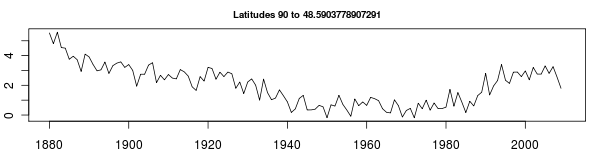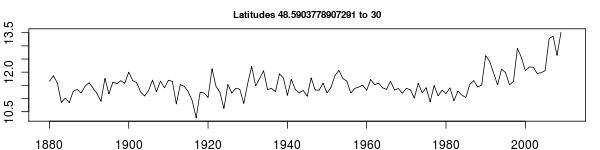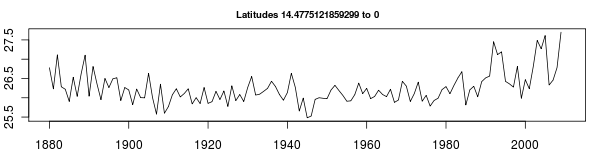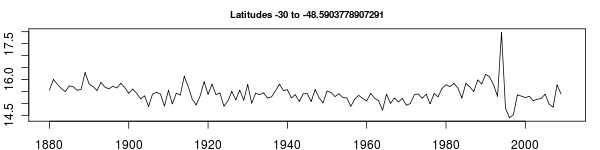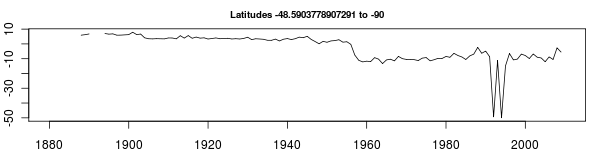1. 2010 July 13 at 7:54 pm

You might think about putting a disclaimer about pre-1973 coverage with each post. Some of these panels are just ugly; somebody coming along might not realise those quirks of GSOD.

Also, that’s a lot of smearing at high latitudes. If I had a vote, I’d vote for GISS-type boxes, but you’ve already said you don’t prefer that sort of thing. Ah well.

2. 2010 July 13 at 8:24 pm

CE, this is all GHCN data.

I’m not sure what you mean by GISS-type boxes.
If you mean – gridding the latitudinal zones – that is coming tomorrow am.

Currently running 36 lat bands x 72 lon bars = 2592 equal area grids – although I’m not sure that the run will be complete by tomorrow am. A 8 bands x 16 bars grid takes about 1.5 hours on my current setup. If this scales directly, it might take 20 hours to run.

3. 2010 July 13 at 8:26 pm

And yeah, I see the odd spikes up and down, especially in this decade. I will track those down, but not before I get the code to a working prototype.

4. 2010 July 13 at 8:42 pm

GISS-type boxes are equal-area grid cells. Sounds like what you are doing.

5. 2010 July 14 at 2:59 am

a glance at the code shows it’s GHCN, yeah, sorry.

by GISS I mean the boxes shown in the 1987 paper. The boxes are of equal area, but not the latitude bands.

6. 2010 July 14 at 3:23 am

oh Ron, downloading the ISH is not fun. I started and gave up. Way too much data. So good luck getting access, and then dealing with it.